# RS Aggarwal Solutions for Class 7 Maths Exercise 15D Chapter 15 Properties of Triangles

RS Aggarwal Solutions for Class 7 Maths Exercise 15D Chapter 15 Properties of Triangles. Subject experts prepare these solutions for properties of triangles to help students for their exam preparations. RS Aggarwal Solutions for Class 7 Maths Chapter 15 Properties of Triangles are available for free download PDF format. Students can either practice online or download these files and practice different types of questions. Questions on Pythagoras theorem and converse of Pythagoras theorem are covered in this exercise of RS Aggarwal Solutions for Class 7 Maths Chapter 15.

## Download the PDF of RS Aggarwal Solutions For Class 7 Maths Chapter 15 Properties of Triangles – Exercise 15D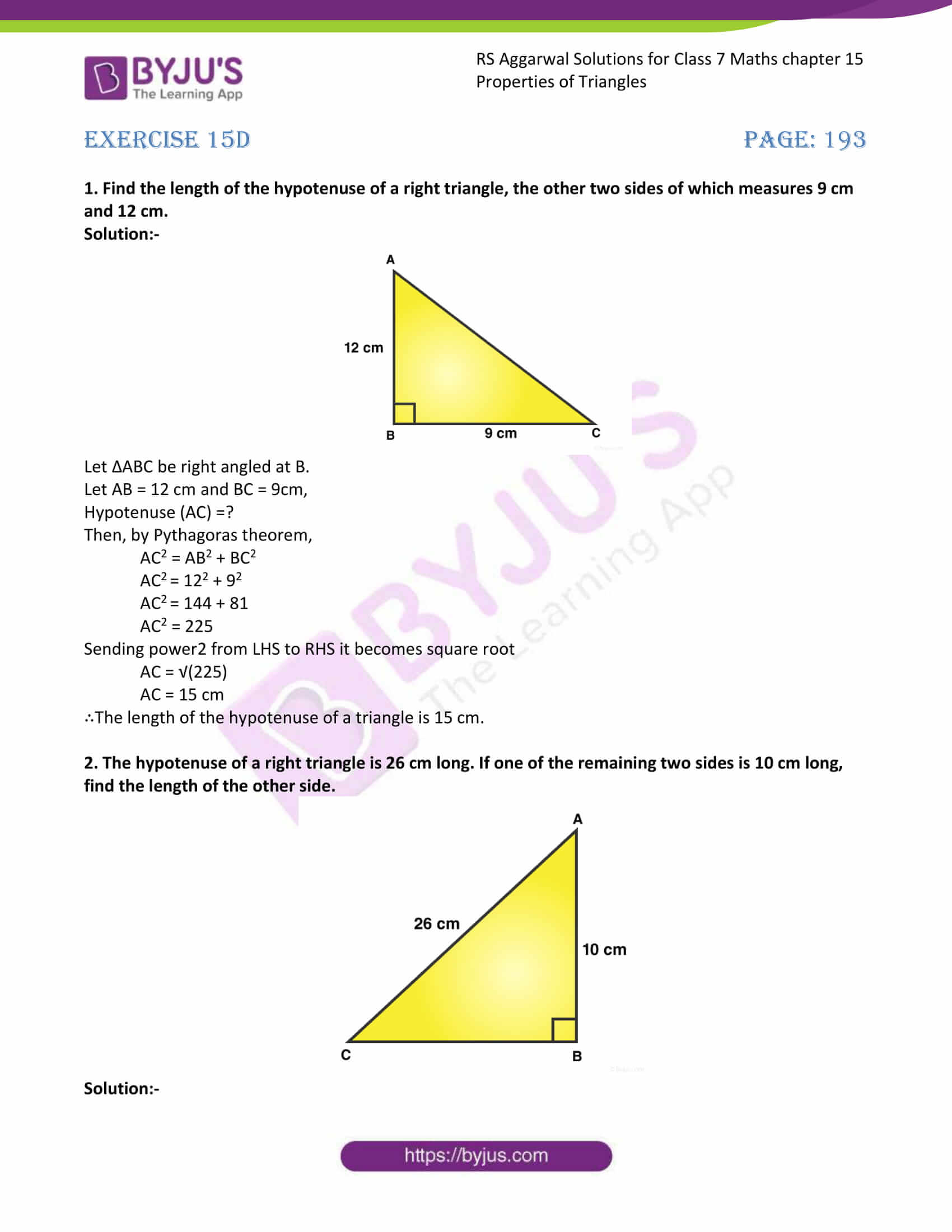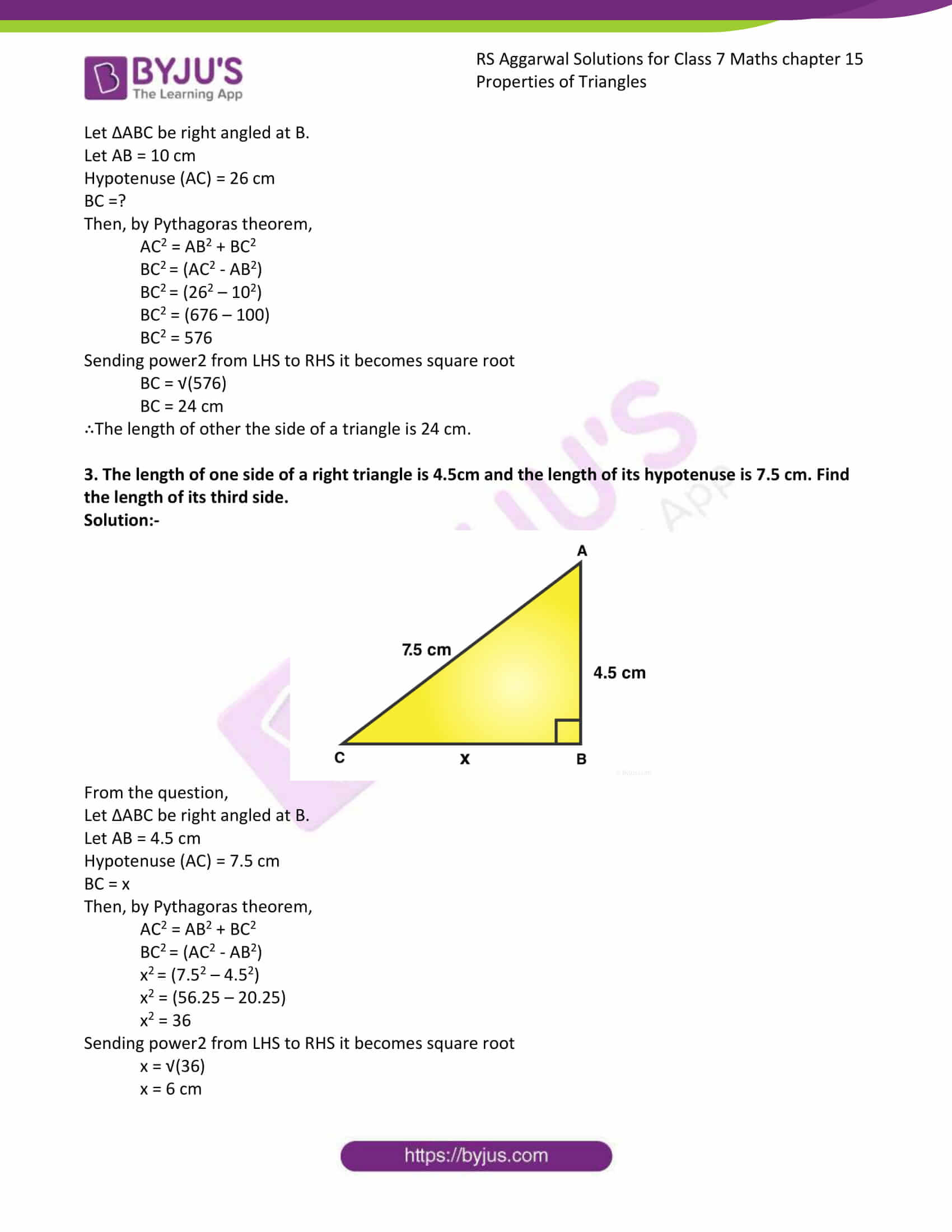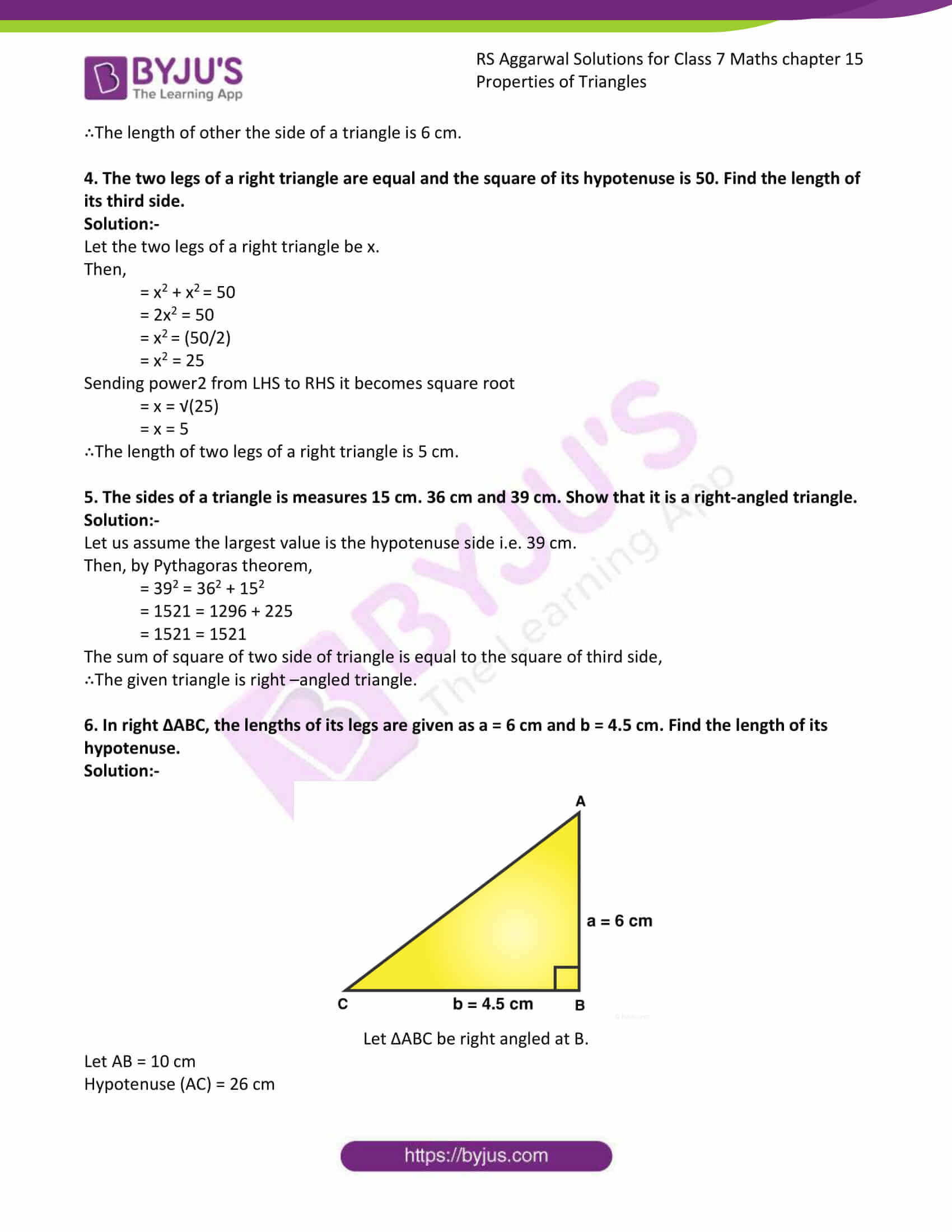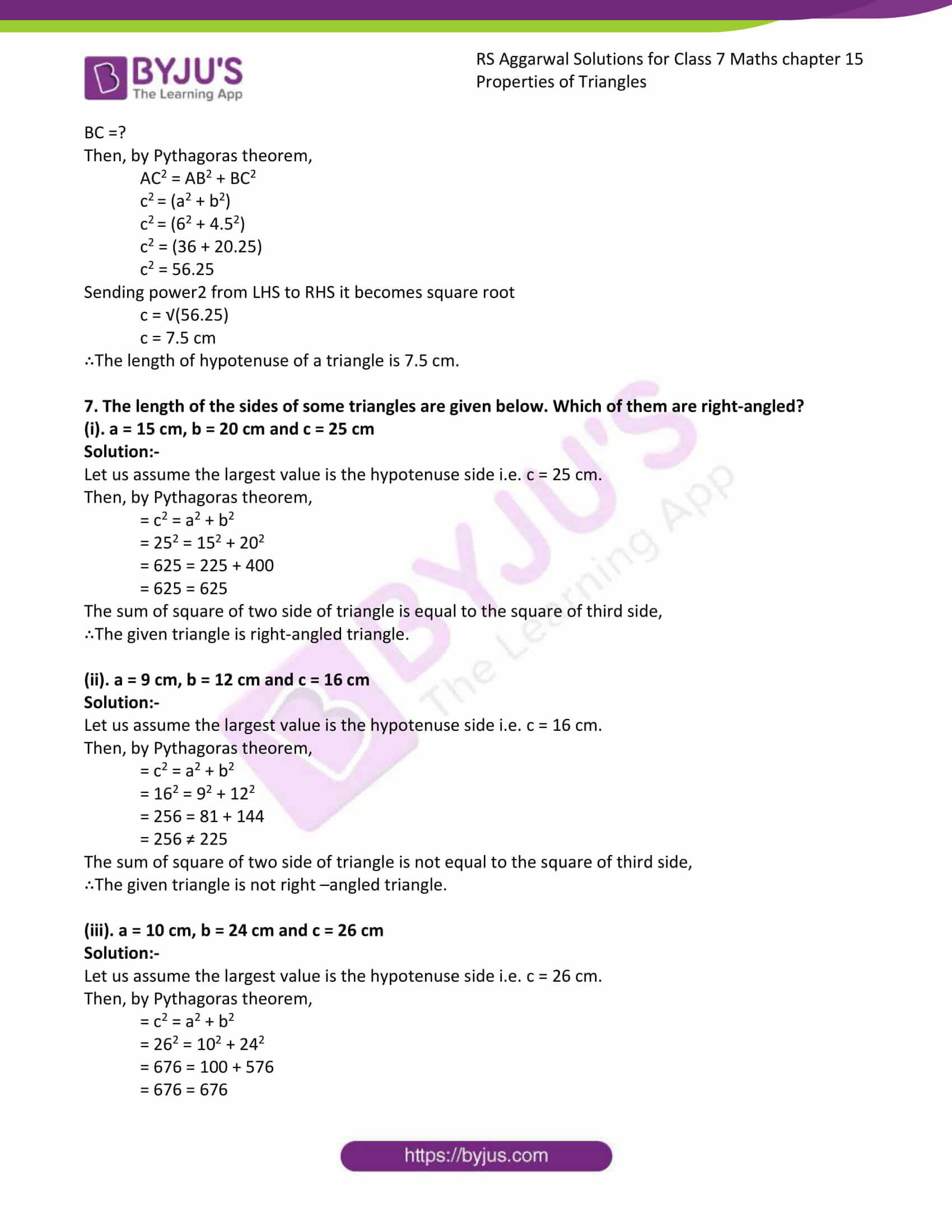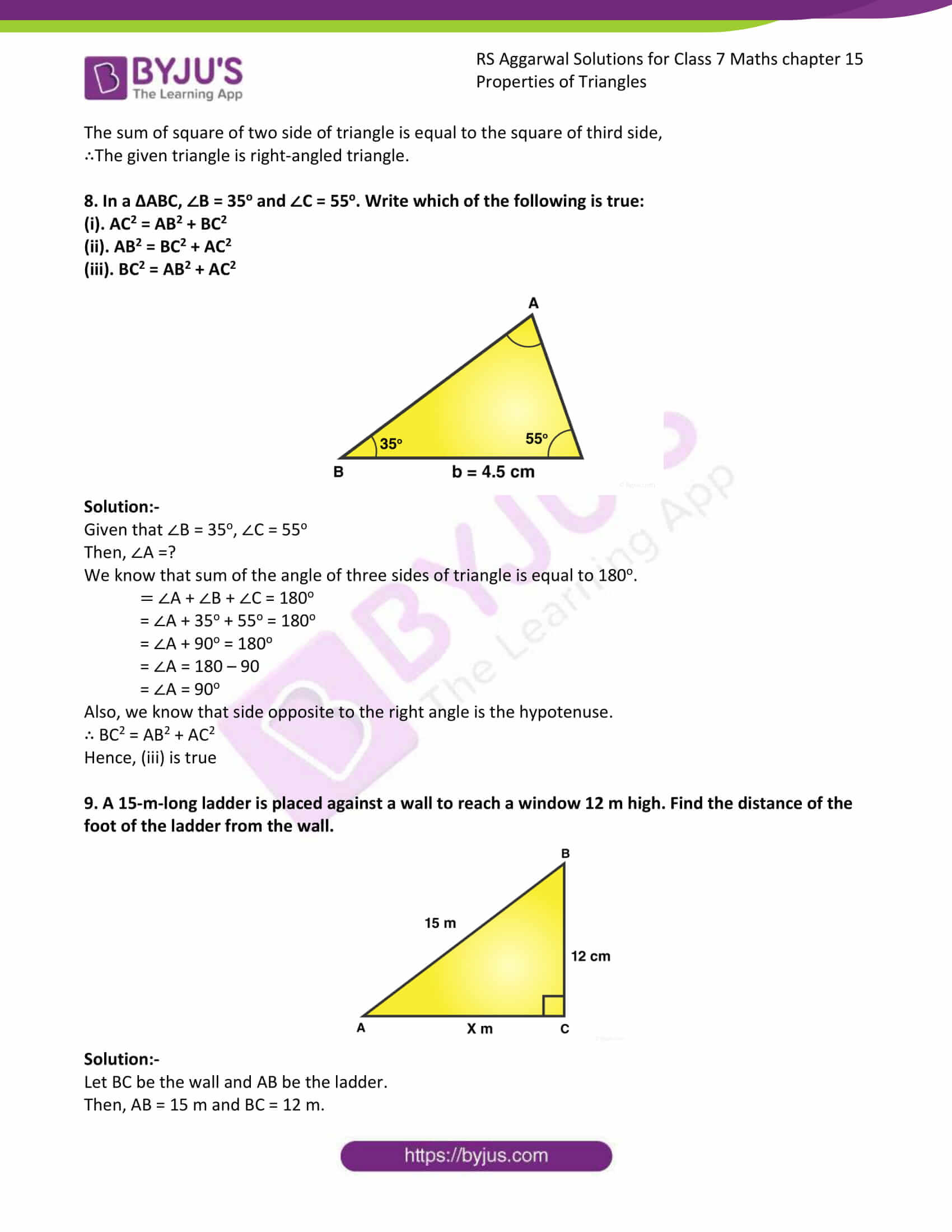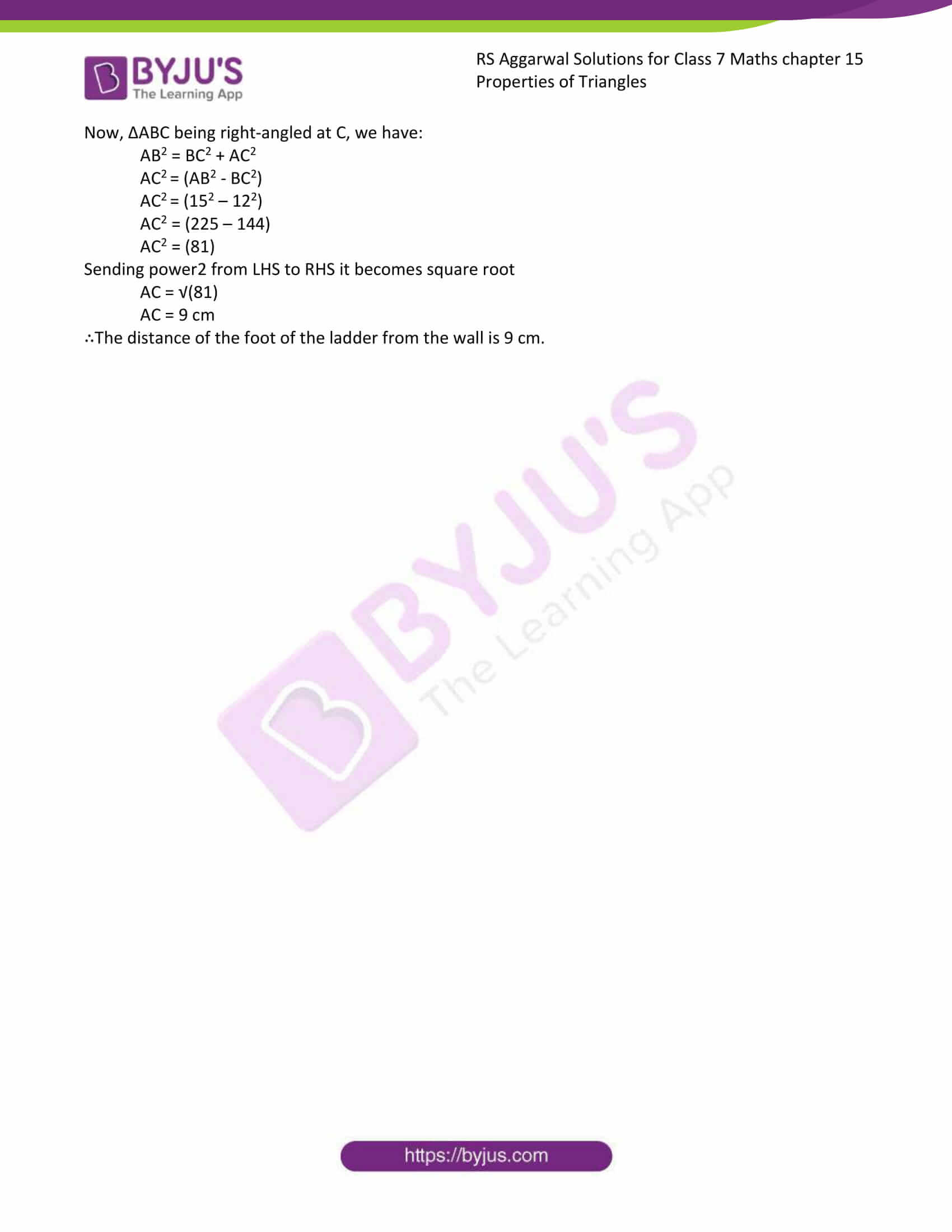### Access answers to Maths RS Aggarwal Solutions for Class 7 Chapter 15 – Properties of Triangles Exercise 15D

1. Find the length of the hypotenuse of a right triangle, the other two sides of which measures 9 cm and 12 cm.

Solution:-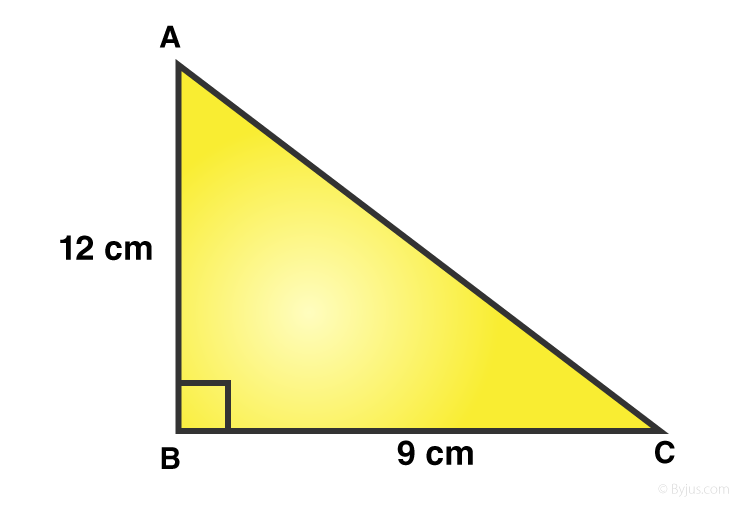Let ΔABC be right angled at B.

Let AB = 12 cm and BC = 9cm,

Hypotenuse (AC) =?

Then, by Pythagoras theorem,

AC2 = AB2 + BC2

AC2 = 122 + 92

AC2 = 144 + 81

AC2 = 225

Sending power2 from LHS to RHS it becomes square root

AC = √(225)

AC = 15 cm

∴The length of the hypotenuse of a triangle is 15 cm.

2. The hypotenuse of a right triangle is 26 cm long. If one of the remaining two sides is 10 cm long, find the length of the other side.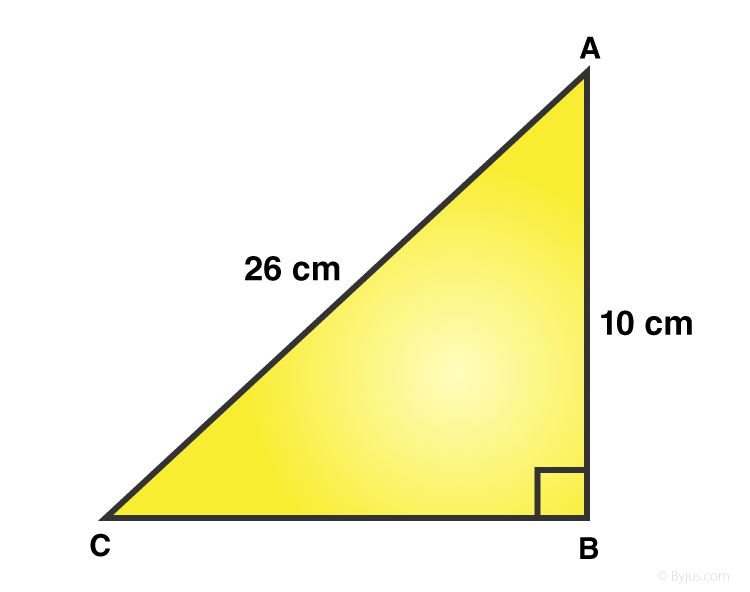Solution:-

Let ΔABC be right angled at B.

Let AB = 10 cm

Hypotenuse (AC) = 26 cm

BC =?

Then, by Pythagoras theorem,

AC2 = AB2 + BC2

BC2 = (AC2 – AB2)

BC2 = (262 – 102)

BC2 = (676 – 100)

BC2 = 576

Sending power2 from LHS to RHS it becomes square root

BC = √(576)

BC = 24 cm

∴The length of other the side of a triangle is 24 cm.

3. The length of one side of a right triangle is 4.5cm and the length of its hypotenuse is 7.5 cm. Find the length of its third side.

Solution:-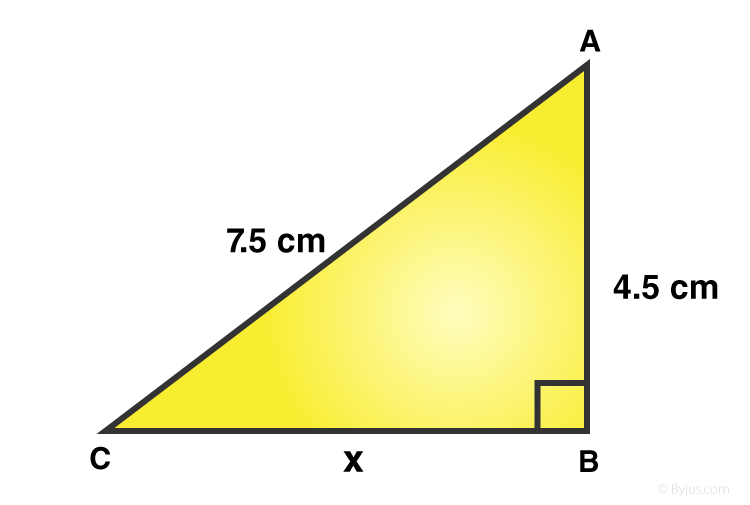From the question,

Let ΔABC be right angled at B.

Let AB = 4.5 cm

Hypotenuse (AC) = 7.5 cm

BC = x

Then, by Pythagoras theorem,

AC2 = AB2 + BC2

BC2 = (AC2 – AB2)

x2 = (7.52 – 4.52)

x2 = (56.25 – 20.25)

x2 = 36

Sending power2 from LHS to RHS it becomes square root

x = √(36)

x = 6 cm

∴The length of other the side of a triangle is 6 cm.

4. The two legs of a right triangle are equal and the square of its hypotenuse is 50. Find the length of its third side.

Solution:-

Let the two legs of a right triangle be x.

Then,

= x2 + x2 = 50

= 2x2 = 50

= x2 = (50/2)

= x2 = 25

Sending power2 from LHS to RHS it becomes square root

= x = √(25)

= x = 5

∴The length of two legs of a right triangle is 5 cm.

5. The sides of a triangle is measures 15 cm. 36 cm and 39 cm. Show that it is a right-angled triangle.

Solution:-

Let us assume the largest value is the hypotenuse side i.e. 39 cm.

Then, by Pythagoras theorem,

= 392 = 362 + 152

= 1521 = 1296 + 225

= 1521 = 1521

The sum of square of two side of triangle is equal to the square of third side,

∴The given triangle is right –angled triangle.

6. In right ΔABC, the lengths of its legs are given as a = 6 cm and b = 4.5 cm. Find the length of its hypotenuse.

Solution:-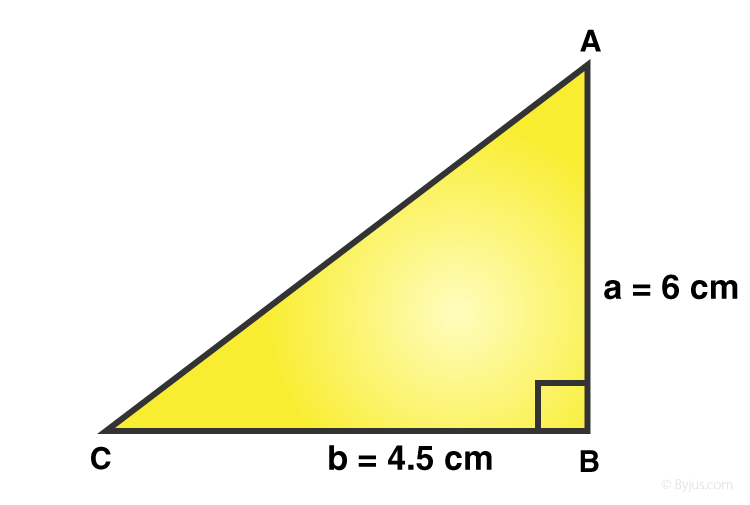Let ΔABC be right angled at B.

Let AB = 10 cm

Hypotenuse (AC) = 26 cm

BC =?

Then, by Pythagoras theorem,

AC2 = AB2 + BC2

c2 = (a2 + b2)

c2 = (62 + 4.52)

c2 = (36 + 20.25)

c2 = 56.25

Sending power2 from LHS to RHS it becomes square root

c = √(56.25)

c = 7.5 cm

∴The length of hypotenuse of a triangle is 7.5 cm.

7. The length of the sides of some triangles are given below. Which of them are right-angled?

(i). a = 15 cm, b = 20 cm and c = 25 cm

Solution:-

Let us assume the largest value is the hypotenuse side i.e. c = 25 cm.

Then, by Pythagoras theorem,

= c2 = a2 + b2

= 252 = 152 + 202

= 625 = 225 + 400

= 625 = 625

The sum of square of two side of triangle is equal to the square of third side,

∴The given triangle is right-angled triangle.

(ii). a = 9 cm, b = 12 cm and c = 16 cm

Solution:-

Let us assume the largest value is the hypotenuse side i.e. c = 16 cm.

Then, by Pythagoras theorem,

= c2 = a2 + b2

= 162 = 92 + 122

= 256 = 81 + 144

= 256 ≠ 225

The sum of square of two side of triangle is not equal to the square of third side,

∴The given triangle is not right –angled triangle.

(iii). a = 10 cm, b = 24 cm and c = 26 cm

Solution:-

Let us assume the largest value is the hypotenuse side i.e. c = 26 cm.

Then, by Pythagoras theorem,

= c2 = a2 + b2

= 262 = 102 + 242

= 676 = 100 + 576

= 676 = 676

The sum of square of two side of triangle is equal to the square of third side,

∴The given triangle is right-angled triangle.

8. In a ΔABC, ∠B = 35o and ∠C = 55o. Write which of the following is true:

(i). AC2 = AB2 + BC2

(ii). AB2 = BC2 + AC2

(iii). BC2 = AB2 + AC2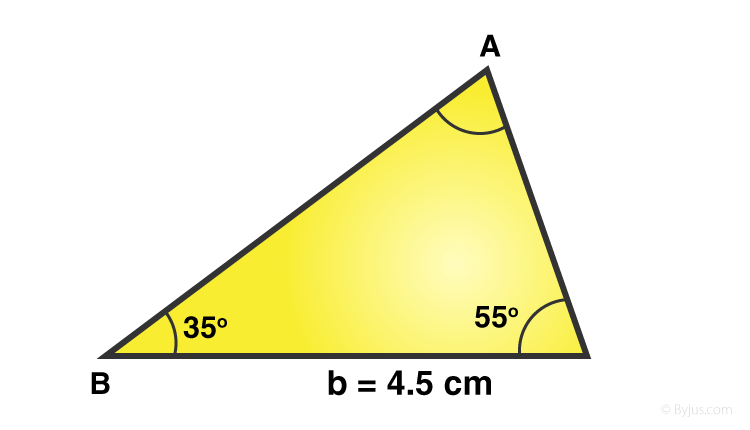Solution:-

Given that ∠B = 35o, ∠C = 55o

Then, ∠A =?

We know that sum of the angle of three sides of triangle is equal to 180o.

= ∠A + ∠B + ∠C = 180o

= ∠A + 35o + 55o = 180o

= ∠A + 90o = 180o

= ∠A = 180 – 90

= ∠A = 90o

Also, we know that side opposite to the right angle is the hypotenuse.

∴ BC2 = AB2 + AC2

Hence, (iii) is true

9. A 15-m-long ladder is placed against a wall to reach a window 12 m high. Find the distance of the foot of the ladder from the wall.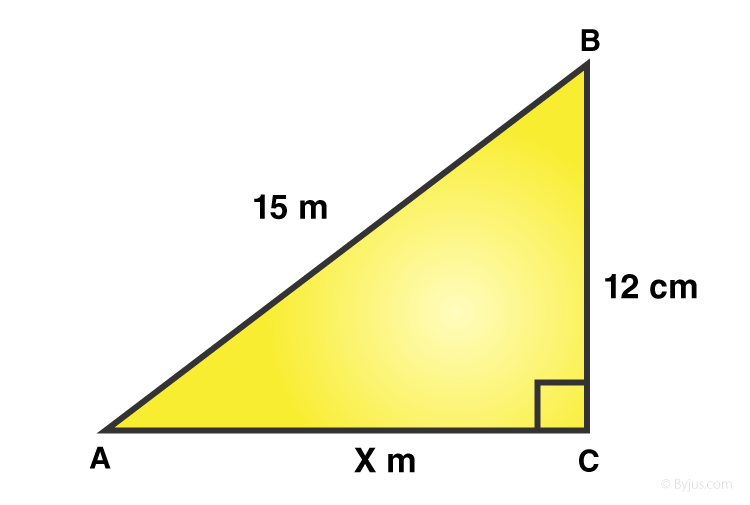Solution:-

Let BC be the wall and AB be the ladder.

Then, AB = 15 m and BC = 12 m.

Now, ΔABC being right-angled at C, we have:

AB2 = BC2 + AC2

AC2 = (AB2 – BC2)

AC2 = (152 – 122)

AC2 = (225 – 144)

AC2 = (81)

Sending power2 from LHS to RHS it becomes square root

AC = √(81)

AC = 9 cm

∴The distance of the foot of the ladder from the wall is 9 cm.

### Access other exercises of RS Aggarwal Solutions For Class 7 Chapter 15 – Properties of Triangles

Exercise 15A Solutions

Exercise 15B Solutions

Exercise 15C Solution